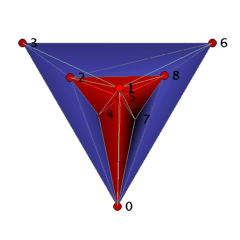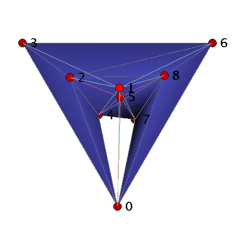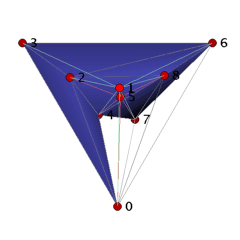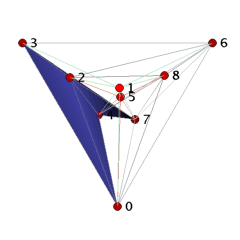A Vertex-Minimal Non-Shellable Simplicial 3-Ball with 9 Vertices and 18 FacetsElectronic Geometry Model No. 2003.05.004

Frank H. Lutz

Description

We present a vertex-minimal non-shellable simplicial 3-ball and hereby answer a question of Danaraj and Klee [8, p. 40].

The concept of shellability plays an important role in the theory of convex polytopes as well as in topology, combinatorics, and computational geometry; see Ziegler  for a survey and further references.

A shelling of a triangulated ball or sphere is a linear ordering of its m facets F_1,...,F_m such that if we remove the facets from the ball or sphere in this order, then at every intermediate step the remaining simplicial complex is a simplicial ball. A simplicial ball or sphere is shellable if it has a shelling; it is extendably shellable if any partial shelling F_1,...,F_i, i<m, can be extended to a shelling. (For a general definition of shellability of simplicial complexes and cell complexes see , , and [21, Ch. 8].)

Two-dimensional balls and spheres are shellable . The first known example of a non-shellable cellular 3-ball is due to Furch and appeared in 1924 . A non-shellable simplicial 3-ball with 30 vertices and 72 facets was provided by Newman in 1926 . Newman's ball is strongly non-shellable, i.e., it has no free facet that can be removed from the triangulation without loosing ballness.

Much smaller strongly non-shellable simplicial 3-balls were obtained by Grünbaum (cf. ) with 14 vertices and 29 facets and by Ziegler  with 10 vertices and 21 facets. Rudin's 3-ball  with 14 vertices and 41 tetrahedra gives a strongly non-shellable rectilinear triangulation of a tetrahedron with all the vertices on the boundary; the vertices even can be moved slightly to yield a straight triangulation of a convex 3-polytope with 14 vertices . Ziegler's ball is realizable as a straight, yet non-convex ball in 3-space. Coordinates for a rectilinear realization of Grünbaum's ball can be found in .

Danaraj and Klee asked in [8, p. 40] ``what is the minimum number of vertices, and of facets, for an unshellable 3-ball''? Triangulated 3-balls with n≤9 vertices were enumerated and tested for shellability in . There is no non-shellable 3-ball with n≤8 vertices, from which it follows that any partial shelling of a 3-ball with n≤8 vertices can be extended to a shelling.

Theorem 1: All triangulated 3-balls with n≤8 vertices are extendably shellable.

Theorem 2: There are precisely twenty-nine vertex-minimal non-shellable simplicial 3-balls with 9 vertices, ten of which are strongly non-shellable. The twenty-nine balls have between 18 and 22 facets, with one unique ball B_3_9_18 having 18 facets and f-vector f=(9,33,43,18).

The ball B_3_9_18 can be visualized as a straight, non-convex ball as follows. We start with the facets 0234 and 2347 to which we add in two steps the facets 2367, 2467, 2468 and 4678, 0678. These 7 tetrahedra form a collar for which we close the hole by gluing in 5 triangles 045, 245, 258, 578, and 057. Finally, we place vertex 1 ``above'' the 11 triangles 023, 024, 045, 057, 068, 078, 236, 245, 258, 268, 578, and we add the cone over the triangles with apex 1 to the complex. The 18 tetrahedra together form a 3-ball with one combinatorial symmetry (0,4)(1,2)(3,5)(6,8). It is easy to check that if we remove any tetrahedron, then we loose the ballness.

Conjecture: The ball B_3_9_18 has the smallest number of facets that a non-shellable simplicial 3-ball can have.

If we take the cone over a strongly non-shellable simplicial d-ball with respect to a new vertex, then the resulting simplicial complex is clearly a strongly non-shellable (d+1)-ball with the same number of facets.

Corollary: There are strongly non-shellable simplicial d-balls with d+6 vertices and 18 facets for d≥3.

Klee and Kleinschmidt  showed that all simplicial d-balls with up to d+3 vertices are (vertex-decomposable and therefore) shellable. (Not vertex-decomposable d-balls with d+4 vertices, d≥3, are given in .) Hence, it remains open whether non-shellable simplicial d-balls with d+4 or d+5 vertices or with fewer than 18 facets exist in dimensions d≥4.

We remark that non-shellable cellular 3-spheres were constructed first by Vince  and by Armentrout . Lickorish  described non-shellable triangulated 3-spheres that contain a knotted triangle made of the sum of (at least) three trefoil knots; suspensions of such spheres produce non-shellable simplicial PL d-spheres in dimensions d≥4. Hachimori and Ziegler  strengthened the result of Lickorish by showing that a triangulated 3-ball or 3-sphere that contains any knotted triangle is (not constructible and thus) non-shellable. An explicit non-shellable triangulated 3-sphere with f-vector f=(381,2309,3856,1928) based on Furch's 3-ball with a knotted spanning arc consisting of one edge was constructed by Hachimori . Examples of non-PL (and hence non-shellable) d-spheres, d≥5, with d+13 vertices can be found in ; see also . However, the smallest known non-shellable 3-sphere, constructed by the author , has only 13 vertices, f-vector f=(13,69,112,56), and is obtained from a non-constructible 12-vertex 3-ball that contains a trefoil knot consisting of three edges; this sphere gives rise to non-shellable simplicial PL d-spheres with d+10 vertices for d≥3.

 Keywords shellability; simplicial balls and spheres; constructibility; vertex-decomposability; PL and non-PL triangulations MSC-2000 Classification 52B22 (52B05, 57Q15) Zentralblatt No. 05264896

References

1. S. Armentrout: Knots and shellable cell partitionings of S^3, Ill. J. Math. 38 (1994), 347-365.
2. R. H. Bing: Some aspects of the topology of 3-manifolds related to the Poincaré conjecture, in T. L. Saaty (Eds.): Lectures on Modern Mathematics, Volume II, John Wiley & Sons (1964), 93-128.
3. A. Björner: Topological methods, in R. Graham, M. Grötschel, and L. Lovász (Eds.): Handbook of Combinatorics, Elsevier (1995), 1819-1872.
4. A. Björner and F. H. Lutz: Simplicial manifolds, bistellar flips and a 16-vertex triangulation of the Poincaré homology 3-sphere, Exp. Math. 9 (2000), 275-289.
5. A. Björner and F. H. Lutz: A 16-vertex triangulation of the Poincaré homology 3-sphere and non-PL spheres with few vertices, Electronic Geometry Models (2004), 2003.04.001, http://www.eg-models.de/2003.04.001.
6. J. Bokowski, D. Bremner, F. H. Lutz, and A. Martin: Combinatorial 3-manifolds with 10 vertices, in preparation.
7. R. Connelly and D. W. Henderson: A convex 3-complex not simplicially isomorphic to a strictly convex complex, Math. Proc. Camb. Phil. Soc. 88 (1980), 299-306.
8. G. Danaraj and V. Klee: Shellings of spheres and polytopes, Duke Math. J. 41 (1974), 443-451.
9. R. Furch: Zur Grundlegung der kombinatorischen Topologie, Abh. Math. Sem. Univ. Hamburg 3 (1924), 69-88.
10. M. Hachimori: Nonconstructible simplicial balls and a way of testing constructibility, Discrete Comput. Geom. 22 (1999), 223-230.
11. M. Hachimori: A 3-sphere with a knotted triangle, http://infoshako.sk.tsukuba.ac.jp/~hachi/math/library/nc_sphere_eng.html.
12. M. Hachimori and G. M. Ziegler: Decompositions of simplicial balls and spheres with knots consisting of few edges, Math. Z. 235 (2000), 159-171.
13. V. Klee and P. Kleinschmidt: The d-step conjecture and its relatives, Math. Oper. Res. 12 (1987), 718-755.
14. W. B. R. Lickorish: Unshellable triangulations of spheres, Eur. J. Comb. 12 (1991), 527-530.
15. F. H. Lutz: Small examples of non-constructible simplicial balls and spheres, SIAM J. Discrete Math., to appear.
16. F. H. Lutz: Vertex-minimal not vertex-decomposable balls, Electronic Geometry Models (2004), 2003.06.001, http://www.eg-models.de/2003.06.001.
17. M. H. A. Newman: A property of 2-dimensional elements, Proc. Royal Acad. Amsterdam 29 (1926), 1401-1405.
18. M. E. Rudin: An unshellable triangulation of a tetrahedron, Bull. Am. Math. Soc. 64 (1958), 90-91.
19. A. Vince: A non-shellable 3-sphere, Eur. J. Comb. 6 (1985), 91-100.
20. G. M. Ziegler: Shelling polyhedral 3-balls and 4-polytopes, Discrete Comput. Geom. 19 (1998), 159-174.
21. G. M. Ziegler: Lectures on Polytopes, Springer-Verlag, Graduate Texts in Mathematics 152 (1995; revised edition, 1998).

Submission information

Submitted: Thu May 29 16:38:08 GMT 2003.
Revised: Tue Mar 16 16:29:57 CET 2004.
Accepted: Sun Mar 21 11:07:26 CET 2004.